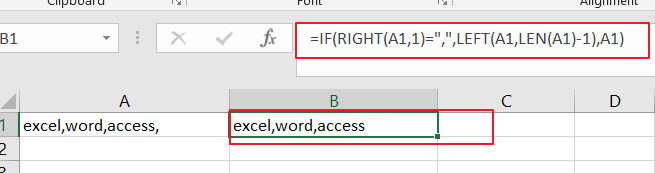# How to Remove Last or Trailing Commas in Excel

This post will guide you how to remove trailing commas from cell in Excel. How do I remove comma character at the end of a cell with a formula in Excel 2013/2016.

## Remove Trailing Commas

if you want to remove trailing commas from you Excel cells, and you can create a formula to check for a comma at the end of a string and then removes the final comma character. you can use the IF function, the RIGHT function, the LEFT function and the LEN function to remove the commas from each cell, like this:

=IF(RIGHT(A1,1)=”,”,LEFT(A1,LEN(A1)-1),A1)

You need to type this formula into a blank cell and press Enter key on your keyboard, and then drag the AutoFill handle over to the range of cells that you want to apply this formula.### Related Functions

• Excel IF function
The Excel IF function perform a logical test to return one value if the condition is TRUE and return another value if the condition is FALSE. The IF function is a build-in function in Microsoft Excel and it is categorized as a Logical Function.The syntax of the IF function is as below:= IF (condition, [true_value], [false_value])….
• Excel LEFT function
The Excel LEFT function returns a substring (a specified number of the characters) from a text string, starting from the leftmost character.The LEFT function is a build-in function in Microsoft Excel and it is categorized as a Text Function.The syntax of the LEFT function is as below:= LEFT(text,[num_chars])…t)…
• Excel RIGHT function
The Excel RIGHT function returns a substring (a specified number of the characters) from a text string, starting from the rightmost character.The syntax of the RIGHT function is as below:= RIGHT (text,[num_chars])…
• Excel LEN function
The Excel LEN function returns the length of a text string (the number of characters in a text string).The syntax of the LEN function is as below:= LEN(text)…